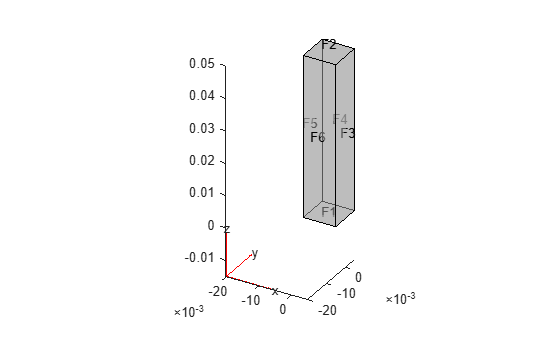# evaluateReaction

Evaluate reaction forces on boundary

## Syntax

``F = evaluateReaction(structuralresults,RegionType,RegionID)``

## Description

example

````F = evaluateReaction(structuralresults,RegionType,RegionID)` evaluates reaction forces on the boundary specified by `RegionType` and `RegionID`. The function uses the global Cartesian coordinate system. For transient and frequency response structural models, `evaluateReaction` evaluates reaction forces for all time- and frequency-steps, respectively.```

## Examples

collapse all

Create a static structural model.

`structuralmodel = createpde('structural','static-solid');`

Create a cuboid geometry and include it in the model. Plot the geometry.

```structuralmodel.Geometry = multicuboid(0.01,0.01,0.05); pdegplot(structuralmodel,'FaceLabels','on','FaceAlpha',0.5);```Specify Young's modulus and Poisson's ratio.

```structuralProperties(structuralmodel,'YoungsModulus',210E9, ... 'PoissonsRatio',0.3);```

Fix one end of the bar and apply pressure to the opposite end.

`structuralBC(structuralmodel,'Face',1,'Constraint','fixed')`
```ans = StructuralBC with properties: RegionType: 'Face' RegionID: 1 Vectorized: 'off' Boundary Constraints and Enforced Displacements Displacement: [] XDisplacement: [] YDisplacement: [] ZDisplacement: [] Constraint: "fixed" Radius: [] Reference: [] Label: [] Boundary Loads Force: [] SurfaceTraction: [] Pressure: [] TranslationalStiffness: [] Label: [] ```
`structuralBoundaryLoad(structuralmodel,'Face',2,'Pressure',100)`
```ans = StructuralBC with properties: RegionType: 'Face' RegionID: 2 Vectorized: 'off' Boundary Constraints and Enforced Displacements Displacement: [] XDisplacement: [] YDisplacement: [] ZDisplacement: [] Constraint: [] Radius: [] Reference: [] Label: [] Boundary Loads Force: [] SurfaceTraction: [] Pressure: 100 TranslationalStiffness: [] Label: [] ```

Generate a mesh and solve the problem.

```generateMesh(structuralmodel,'Hmax',0.003); structuralresults = solve(structuralmodel);```

Compute the reaction forces on the fixed end.

`reaction = evaluateReaction(structuralresults,'Face',1)`
```reaction = struct with fields: Fx: -1.3620e-06 Fy: 2.2303e-06 Fz: 0.0103 ```

Evaluate the reaction forces at the fixed end of a beam subject to harmonic excitation.

Create a transient dynamic model for a 3-D problem.

`structuralmodel = createpde('structural','transient-solid');`

Create a geometry and include it in the model. Plot the geometry.

```gm = multicuboid(0.06,0.005,0.01); structuralmodel.Geometry = gm; pdegplot(structuralmodel,'FaceLabels','on','FaceAlpha',0.5) view(50,20)```Specify Young's modulus, Poisson's ratio, and the mass density of the material.

```structuralProperties(structuralmodel,'YoungsModulus',210E9, ... 'PoissonsRatio',0.3, ... 'MassDensity',7800);```

Fix one end of the beam.

`structuralBC(structuralmodel,'Face',5,'Constraint','fixed');`

Apply a sinusoidal displacement along the y-direction on the end opposite the fixed end of the beam.

```structuralBC(structuralmodel,'Face',3, ... 'YDisplacement',1E-4, ... 'Frequency',50);```

Generate a mesh.

`generateMesh(structuralmodel,'Hmax',0.01);`

Specify the zero initial displacement and velocity.

`structuralIC(structuralmodel,'Displacement',[0;0;0],'Velocity',[0;0;0]);`

Solve the model.

```tlist = 0:0.002:0.2; structuralresults = solve(structuralmodel,tlist);```

Compute the reaction forces on the fixed end.

`reaction = evaluateReaction(structuralresults,'Face',5)`
```reaction = struct with fields: Fx: [101x1 double] Fy: [101x1 double] Fz: [101x1 double] ```

## Input Arguments

collapse all

Solution of the structural analysis problem, specified as a `StaticStructuralResults`, `TransientStructuralResults`, or `FrequencyStructuralResults` object. Create `structuralresults` by using the `solve` function.

Example: ```structuralresults = solve(structuralmodel)```

Geometric region type, specified as `'Edge'` for a 2-D model or `'Face'` for a 3-D model.

Example: `evaluateReaction(structuralresults,'Face',2)`

Data Types: `char` | `string`

Geometric region ID, specified as a vector of positive integers. Find the region IDs by using `pdegplot`.

Example: `evaluateReaction(structuralresults,'Face',2)`

Data Types: `double`

## Output Arguments

collapse all

Reaction forces, returned as a structure array. The array fields represent the integrated reaction forces and surface traction vector, which are computed by using the state of stress on the boundary and the outward normal.

## Version History

Introduced in R2017b